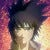# Face Mask Detection using Faster RCNN

Faster RCNN is an efficient tool for detecting objects in 2D color images. The model was first proposed in TPAMI 2016, and is an improvement over previous works of RCNN and Fast RCNN, by introducing deep regional proposal networks.

# A Quick Example Using LSTM in Stock Market Prediction

LSTM is a very convenient tool for making time-series predictions, so it’s not surprising that it could be used for stock market estimation. Here we give a quick demo for building a 2-layer stateless LSTM for Nasdaq index prediction, which is adapted from this Kaggle version, with minor adjustments. …

# Self-attention and the Non-local Network

Self-attention is a concept which has probably been discussed a million times, in the context of the Transformer. On the one hand, the proposal of Transformer solved the problem of modelling long recurrence by the use of attention mechanisms. …

# [Pytorch Learning 101] Basics of PyTorch Tensor, Autograd and Partial Derivatives

For the weekend I was stuck on a simple question: If I have a matrix A¹⁰, how do I use PyTorch to calculate the following:

# Bayesian Optimization Basics (1)

In this article, we will talk about a few basic concepts based on the paper “Practical Bayesian Optimization of Machine Learning Algorithms” published in NIPS 2012.

The content includes the following:

1. Surrogate functions and Gaussian Processes.
2. Acquisition functions.
3. Possible limitations of Bayesian optimization.

Surrogate functions and Gaussian Processes.

What is…

# Hyperparameter-tuning for Deep Learning Models with the Ax

The Ax API (https://ax.dev/) is a nice alternative to the last article I posted, using the Ray for Bayesian optimization.

We still use the same example of MNIST classification network, and see how the usage slightly differs. A quick comparison of the results is given at the end of this…

# Hyperparameter-tuning for Deep Learning Models with the Ray — Simple Pytorch Example